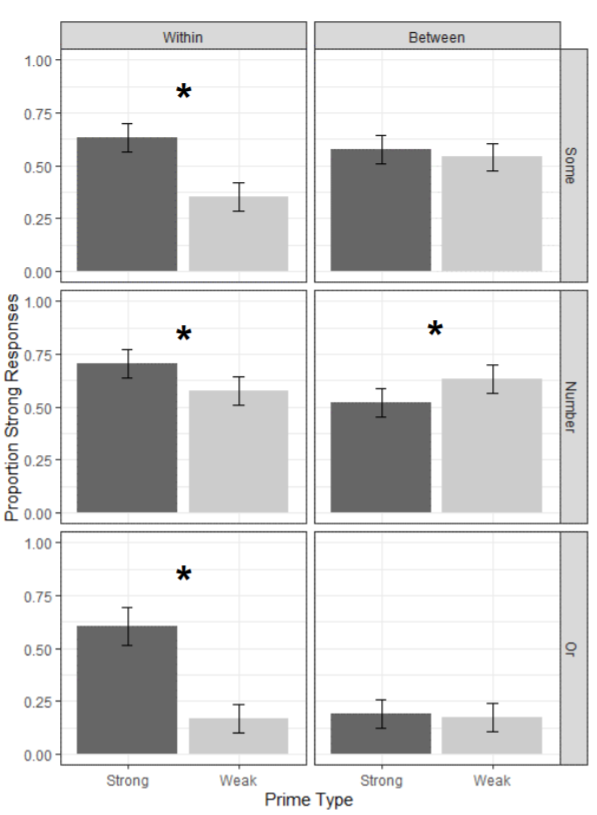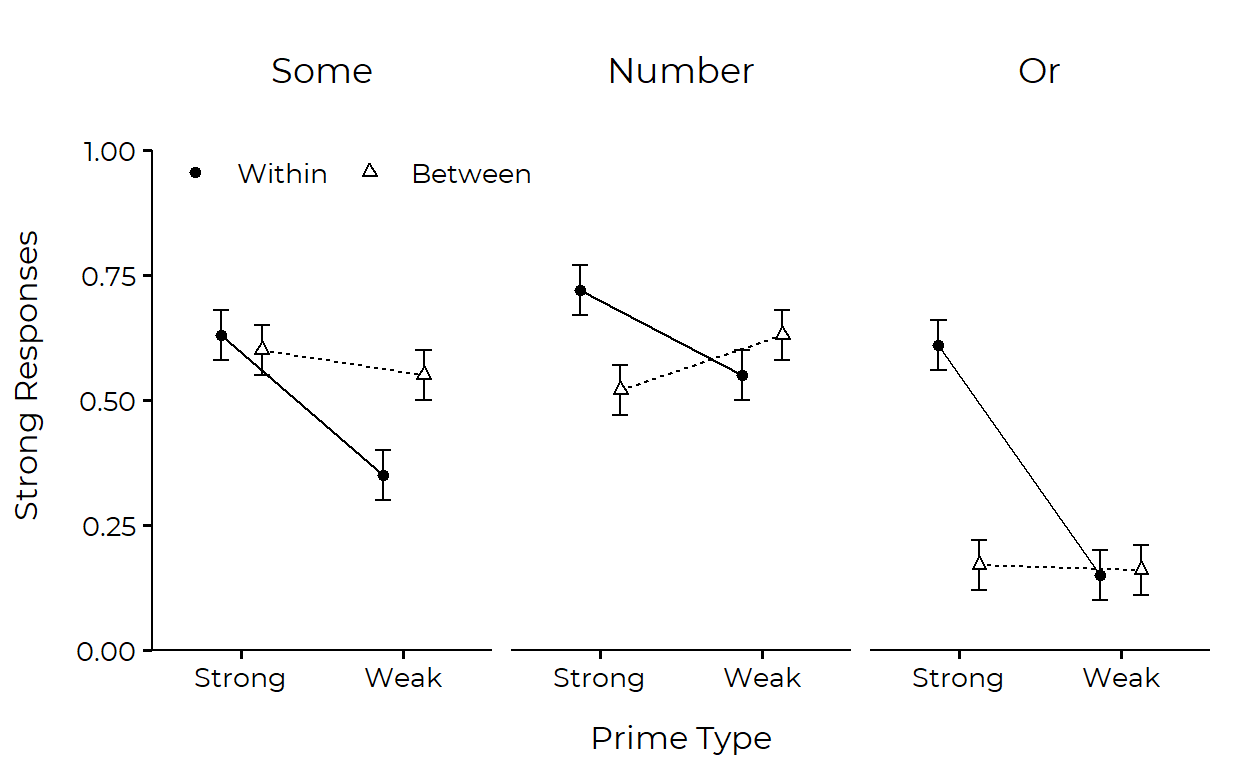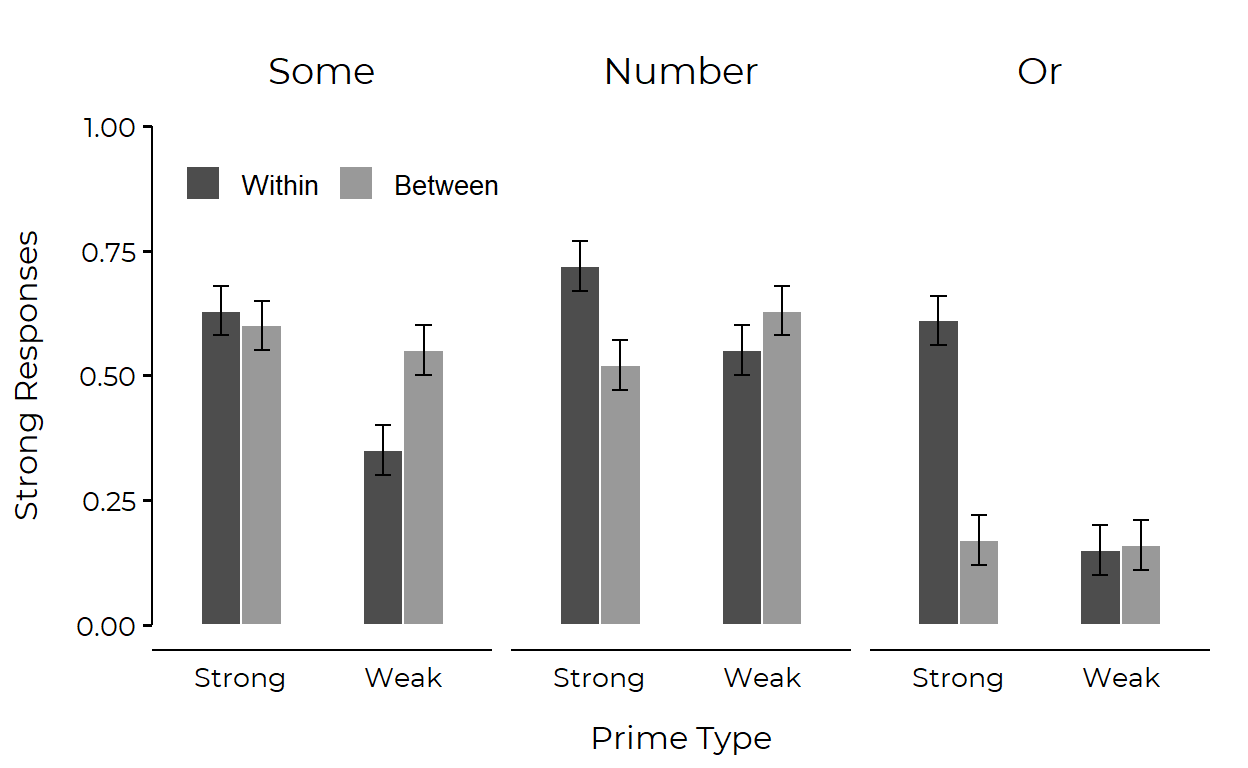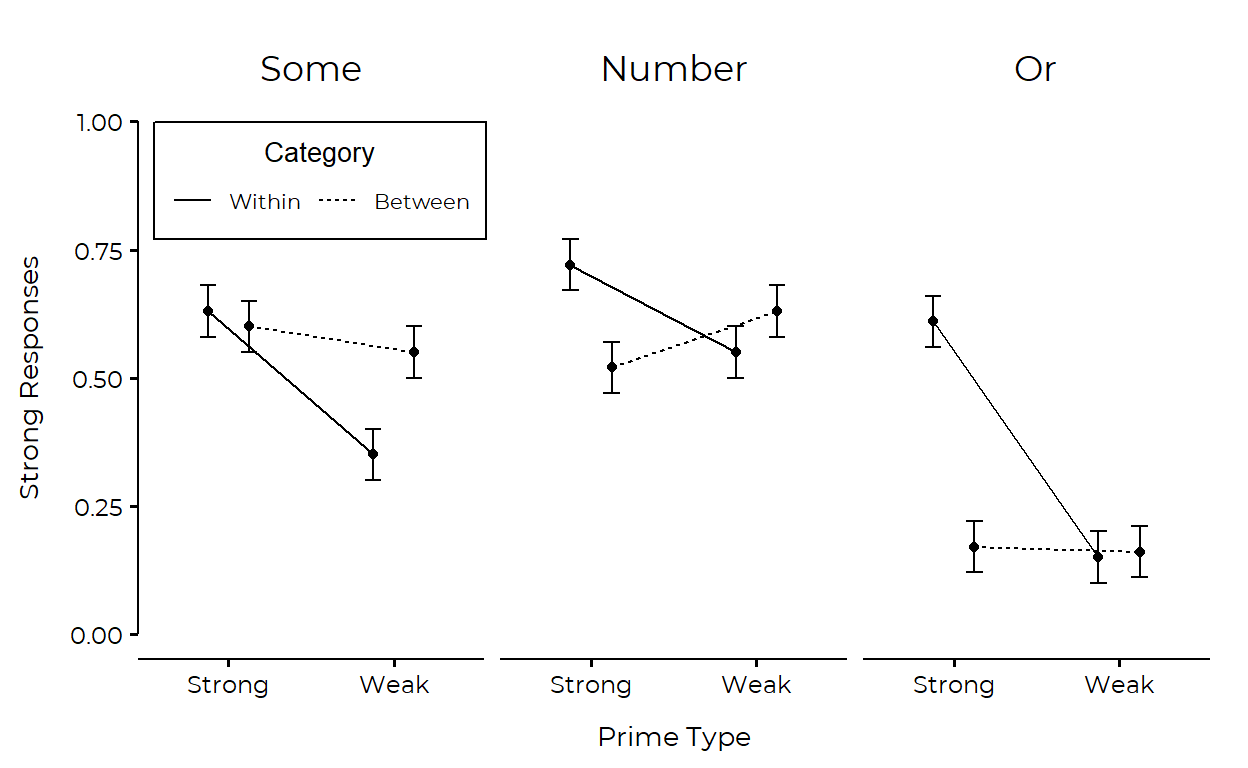# Plot Makeover #1

Flattening a faceted grid for strictly horizontal comparisons

June Choe (University of Pennsylvania Linguistics)https://live-sas-www-ling.pantheon.sas.upenn.edu/
09-20-2020

This is the first installment of plot makeover where I take a plot in the wild and make very opinionated modifications to it.

## Before

Our plot-in-the-wild comes from the recent AMLAP 2020 conference, where I presented my thesis research and had the opportunity to talk with and listen to expert psycholinguists around the world. The plot that I’ll be looking at here is Figure 3 from the abstract of a work by E. Matthew Husband and Nikole Patson .Figure 1: Plot from Husband and Patson (2020)

What we have is 6 pairs of barplots with error bars, laid out in a 2-by-3 grid. The total of 12 bars are grouped at three levels which are mapped in the following way:

• First level is mapped to the grid column.

• Second level is mapped to the grid row.

• Third level is mapped to the x-axis.

To get a better sense of what they did, and to make data for the plot makeover, I have recreated the original plot below:1

1. Data

``````library(tidyverse)
df <- crossing(level_1 = fct_inorder(c("Within", "Between")),
level_2 = fct_inorder(c("Some", "Number", "Or")),
level_3 = factor(c("Strong", "Weak")))
df\$barheight <- c(.63, .35, .72, .55, .61, .15, .60, .55, .52, .63, .17, .16)

df
``````
``````  # A tibble: 12 x 4
level_1 level_2 level_3 barheight
<fct>   <fct>   <fct>       <dbl>
1 Within  Some    Strong       0.63
2 Within  Some    Weak         0.35
3 Within  Number  Strong       0.72
4 Within  Number  Weak         0.55
5 Within  Or      Strong       0.61
6 Within  Or      Weak         0.15
7 Between Some    Strong       0.6
8 Between Some    Weak         0.55
9 Between Number  Strong       0.52
10 Between Number  Weak         0.63
11 Between Or      Strong       0.17
12 Between Or      Weak         0.16``````

2. Plot

``````df %>%
ggplot(aes(level_3, barheight)) +
geom_col(
aes(fill = level_3),
show.legend = FALSE
) +
geom_errorbar(
aes(ymin = barheight - .05, ymax = barheight + .05),
width = .1) +
facet_grid(level_2 ~ level_1) +
theme_bw() +
scale_fill_manual(values = c('grey40', 'grey80')) +
ylim(0, 1) +
labs(
y = "Proportion of Strong Responses",
x = "Prime Type") +
theme_bw()
``````## My Plan

Major Changes:

• Flatten the grid in some way so that everything is laid out left-to-right and you can make comparisons horizontally.

• Cap the y axis to make it clear that the values (proportions) can only lie between 0 and 1.

Minor Changes:

• Remove grid lines

• Increase space between axis and axis titles.

• Remove boxes around strip labels

• Make strip (facet) labels larger and more readable.

• Increase letter spacing (probably by changing font)

## After

I actually couldn’t settle on one final product2 so here are two plots that incorporate the changes that I wanted to make. I think that both look nice and you may prefer one style over the other depending on what relationships/comparisons you want your graph to emphasize.

### Point-line plot

I got a suggestion that the groups could additionally be mapped to shape for greater clarity, so I’ve incorporated that change.3``````dodge <- position_dodge(width = .5)

df %>%
mutate(level_3 = as.numeric(level_3)) %>%
ggplot(aes(x = level_3, y = barheight, group = level_1)) +
geom_errorbar(
aes(ymin = barheight - .05, ymax = barheight + .05),
width = .2,
position = dodge
) +
geom_line(
aes(linetype = level_1),
position = dodge,
show.legend = FALSE
) +
geom_point(
aes(shape = level_1, fill = level_1),
size = 1.5,
stroke = .6,
position = dodge
) +
scale_fill_manual(values = c("black", "white")) +
scale_shape_manual(values = c(21, 24)) +
facet_wrap(~ level_2) +
scale_x_continuous(
breaks = 1:2,
labels = levels(df\$level_3),
expand = expansion(.2),
) +
scale_y_continuous(
limits = c(0, 1),
expand = expansion(c(0, .1))
) +
lemon::coord_capped_cart(left = "both") +
guides(
fill = guide_none(),
shape = guide_legend(
title = NULL,
direction = "horizontal",
label.theme = element_text(size = 10, family = "Montserrat"),
override.aes = list(fill = c("black", "white"))
)
) +
labs(
y = "Strong Responses",
x = "Prime Type",
linetype = "Category"
) +
ggthemes::theme_clean(base_size = 14) +
theme(
text = element_text(family = "Montserrat"),
legend.position = c(.18, .87),
legend.background = element_rect(color = NA, fill = NA),
strip.text = element_text(size = 13),
plot.margin = margin(5, 5, 5, 5, 'mm'),
axis.title.x = element_text(vjust = -3),
axis.title.y = element_text(vjust = 5),
plot.background = element_blank(),
panel.grid.major.y = element_blank()
)
``````

### Bar plot``````dodge <- position_dodge(width = .5)

df %>%
mutate(level_3 = as.numeric(level_3)) %>%
ggplot(aes(x = level_3, y = barheight, group = level_1)) +
geom_col(position = dodge, width = .5, color = 'white', aes(fill = level_1)) +
scale_fill_manual(values = c("grey30", "grey60")) +
geom_errorbar(
aes(ymin = barheight - .05, ymax = barheight + .05),
width = .2,
position = dodge
) +
facet_wrap(~ level_2) +
scale_x_continuous(
breaks = 1:2,
labels = levels(df\$level_3),
expand = expansion(.2),
) +
ylim(0, 1) +
lemon::coord_capped_cart(left = "both") +
labs(
y = "Strong Responses",
x = "Prime Type",
fill = NULL
) +
ggthemes::theme_clean(base_size=14) +
theme(
text = element_text(family = "Montserrat"),
legend.text = element_text(size = 10),
legend.key.size = unit(5, 'mm'),
legend.direction = "horizontal",
legend.position = c(.17, .85),
legend.background = element_blank(),
strip.text = element_text(size = 14),
axis.ticks.x = element_blank(),
axis.title.x = element_text(vjust = -3),
axis.title.y = element_text(vjust = 5),
panel.grid.major.y = element_blank(),
plot.background = element_blank(),
plot.margin = margin(5, 5, 5, 5, 'mm')
)
``````
Husband, E. Matthew, and Nikole Patson. 2020. Priming of Implicatures Within and Between Categories: The Case of or. AMLaP2020. https://amlap2020.github.io/a/272.pdf.

1. But note that this is likely not how the original plot was generated: the authors were likely feeding ggplot2 with the raw data (involving 1s and 0s in this case), but here I am just grabbing the summary statistic that was mapped to the bar aesthetic (hence my decision to name the y variable `barheight`).↩︎

2. I ran the first plot by a friend who has a degree in design, and she recommended several changes that eventually ended up being the second plot. Some major pointers were removing border lines from the legend, removing x-axis tick marks, and applying color/shade.↩︎

3. The plot used to look like this:↩︎